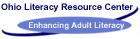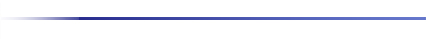You are here - OLRC Home   |   Site Index   |   Contact UsMath Literacy Newsletter: Volume 1 October 1994

The three Ohio delegates to the Adult Mathematical Literacy Conference in Washington last spring have organized a network of adult education math instructors in Ohio. Nancy Marcus, an instructor in Cleveland Heights/University Heights, Shelia Sides-Jones, an instructor in Mansfield City Schools, and Jean Stephens, Director of the Ohio Literacy Resource Center provided the leadership for the initial planning. Over thirty-five instructors from all parts of Ohio have expressed interest in participating in the project, and twenty of them met in mid-September to begin planning math activities.

The instructors expressed interest in developing math training workshops, developing curriculum, arranging visitations, and sharing math ideas through a newsletter. The group also plans to facilitate seven sites for the December mathematics tele-conference. The instructional ideas they shared at the meeting are the basis for this newsletter. The group also spent time discussing the Massachusetts Adult Basic Education Math Standards and the possible adoption of these standards in Ohio.

Paula Mullet - Cleveland Hts. - University Hts. ABLE
The Techniques of Problem Solving (TOPS) Cards produced by Dale Seymour Publications (P.O. Box 10888, Palo Alto, CA 94303) are very useful with students. The sets of 200 cards are available for grade levels 3-9. The cards require students to use a variety of problem-solving strategies to answer questions.

I use the cards as an introductory activity. I copy 2 or 3 questions on the board each morning for people to work on when they arrive. When most students have completed a question, we go over the answers as a class.

Delores Jones - Southwest ABLE/JOBS
Metrics - Use Deci-Mat (double-sided, flat vinyl mat that teaches whole numbers and deimals) to teach place-value and show that the metric system just uses other names for the place-values.

Students should:

1. Know the names of the "places"
2. Know that each of those places represent multiples of 10
3. Know that the "line" on the Deci-Mat or place-value chart, which comes after the "ones" place is where the decimal would be placed
4. Know that each "move" to the left on the place-value chart means that unit is 10 times as big as the unit in the place to its right
5. Know that each "move" to the right on the place-value chart means that unit is one-tenth as big as the unit to its left. Simply write the metric name for each place-value on the Deci-Mat or place-value chart [thousands (kilo-); hundreds (hecto-); tens (deka-); ones or units (meter, gram or liter); tenths (deci-); hundredths (centi-); and thousandths (milli-)]

Carolyn Naples Taylor - Warren City Schools
This lesson introduces circles and the meaning and use of the terms circumference, diameter, and pi.

Materials: Chart paper, markers, scissors, string, compass, card stock, copy paper, or heavy weight construction paper. (You can also use coins and thread or thin string.)

Introduction: Ask students what they know about circles. Record all responses on chart paper. (You will ask this question again at the end of the lesson to compare new knowledge to old.)

Activity: Show students how to use a compass. Have them draw circles of different sizes and cut them out. Stretch string around the circle once, and cut. Have them stretch the string back and forth across the circle, making sure they cross the center. Have them record how many times the string goes across.

Discussion: Ask students what they discovered. Ask them to come up with a rule for circles. Ask if they think there are exceptions. Explain the history of measuring land by using knotted ropes and sectioning off circular plots of land. The rule they just discovered was also discovered back then. Put names to features of the circle that they have been working with and discuss ratio of circumference to diameter to talk about pi. Develop the formula for circumference and diameter.

E. Jean Thom -- Strongsville City Schools ABLE
This is a good introductory activity for both the students and the instructor.

Objectives: The student will (1) develop a sense of what numbers are and how they can be represented in a variety of equivalent forms, (2) understand that integers can be expressed in an unlimited number of ways, and (3) explore and recognize patterns in numbers.

Activity: On a sheet of paper, print your name vertically down the left side of the paper. Find the numerical value of your name using the operation of addition. A=1, B=2, C=3, etc. After you have determined the value of your name, write that value in at least ten different ways. Students may work together if they prefer.

Example:

```E =     5
T =    20
H =     8
E =     5
L =    12
J =    10
E =     5
A =     1
N =    14
T =    20
H =     8
O =    15
M =    13
136```

One-hundred thirty-six
CXXXVI
Patterns:
100 + 36; 99 + 37; 98 + 38...
137 - 1; 138 - 2; 139 - 3 ...
135 +1; 135 + 2/2; 135 + 3/3 ...
135.1 + .9; 135.2 + .8; 135.3 + .7 ...

100 + 36 = 36 + 100

Expanded Notation
100 + 30 + 6
1(100) + 3(10) +6(1)
1(102) + 3(101) + 6(10)

Note: This exercise can be expanded to introduce properties, expanded notation, and scientific notation.

Dorna Smith-- Gallia-Jackson-Vinton JVSD
Utilizing Multi Sensory Learning with Multiplication Facts To incorporate "tactile" practices in multiplication: Encourage students to complete a multiplication chart each day, then refer to the chart as needed for the remainder of the day. The repetition and the writing will reinforce the information.

To incorporate "visual" practices in multiplication: Encourage student to first enter the facts that he/she knows --2's, 5's, 10's, 11's, etc. Enter the facts both horizontally and vertically.

Point out:

``` 1   2   3   4   5    6    7      8    9    10   11    12
2                               16                    24
3                               24                    36
4                               32                    48
5                               40                    60
6                               48                    72
7                               56                    84
8                               64                    96
9                               72                   108
10                               80                   120
11                               88                   132
12                               96                   144```
• How the 11's are the MULTIPLIER "doubled" i.e. 22, 33, 44, etc. until you get to "11x10" and above.
• The multiples of 5's always end in 5 or 0
• The multiples of 10's always end in 0. It's the MULTIPLIER plus zero.
• The visual arrangement of the 8's and the 12's. (Look at the sequence pattern of the right hand number, then look at the pattern of the number on the left.)
• The way to multiply 9's on your fingers.
• How to use flash cards.
• Encourage student to "Beat their own time" in completing the chart. If memorization of these facts is a problem, the student can always "duplicate" the chart in the corner of a test paper.
• To incorporate "Auditory" methods: Have the student explain the above rules to someone else.
• Have the student say the various multiplication tables aloud. Emphasize any rhythm patterns that occur.

Patti Bilyeu -- Belmont-Harrison VSD
Multiplication - Troublesome facts

Most students will master the basic facts up to the 6 tables easily. Below are 3 examples to help with 7x6, 7x8, and 6x8

```7x6 can mean  		7+7+7=21    so      7x3=21  so        21
7+7+7=21            7x3=21           +21
42

7x8 = 56		6x8=48
5678
```This page http://literacy.kent.edu/Oasis/Pubs/math.htm
 Follow us on